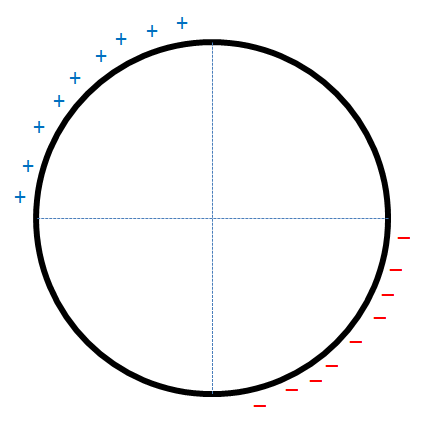# Like a Rolling Stone!The figure shows a non-conducting, non-uniformly charged ring with linear charge densities $\lambda$ and $- \lambda$, mass $m$ and radius $R$. This ring is placed on a rough horizontal surface and a horizontal electric field $\vec{E}$ is switched on.

If at some instant, the ring is in the position shown above and is rolling without slipping, find the minimum coefficient of friction $\mu_{min}$ required.

Details & Assumptions

1. A uniform gravitational field $|\vec{g}| = 10 ms^{-2}$ acts downward.
2. $\lambda = \dfrac{\pi}{100} Cm^{-1}$
3. $m = 1.5 kg$
4. $R = 0.15 m$
5. $|\vec{E}| = 1000 NC^{-1}$
×

Problem Loading...

Note Loading...

Set Loading...